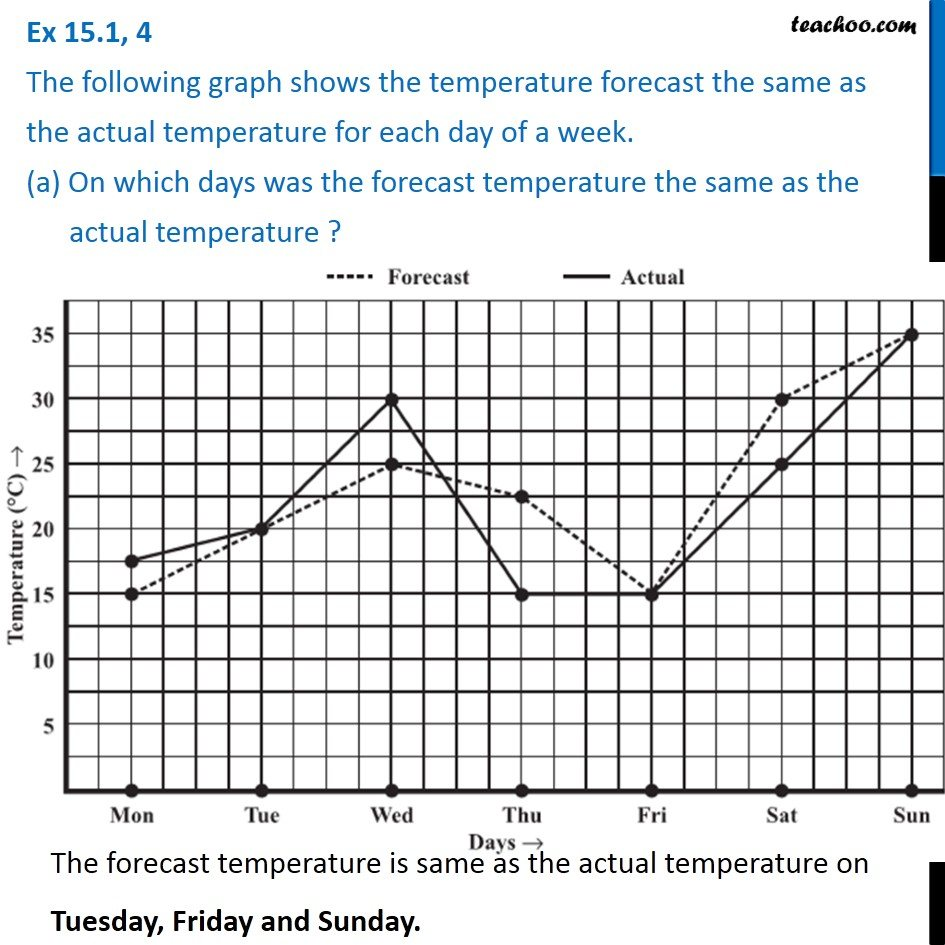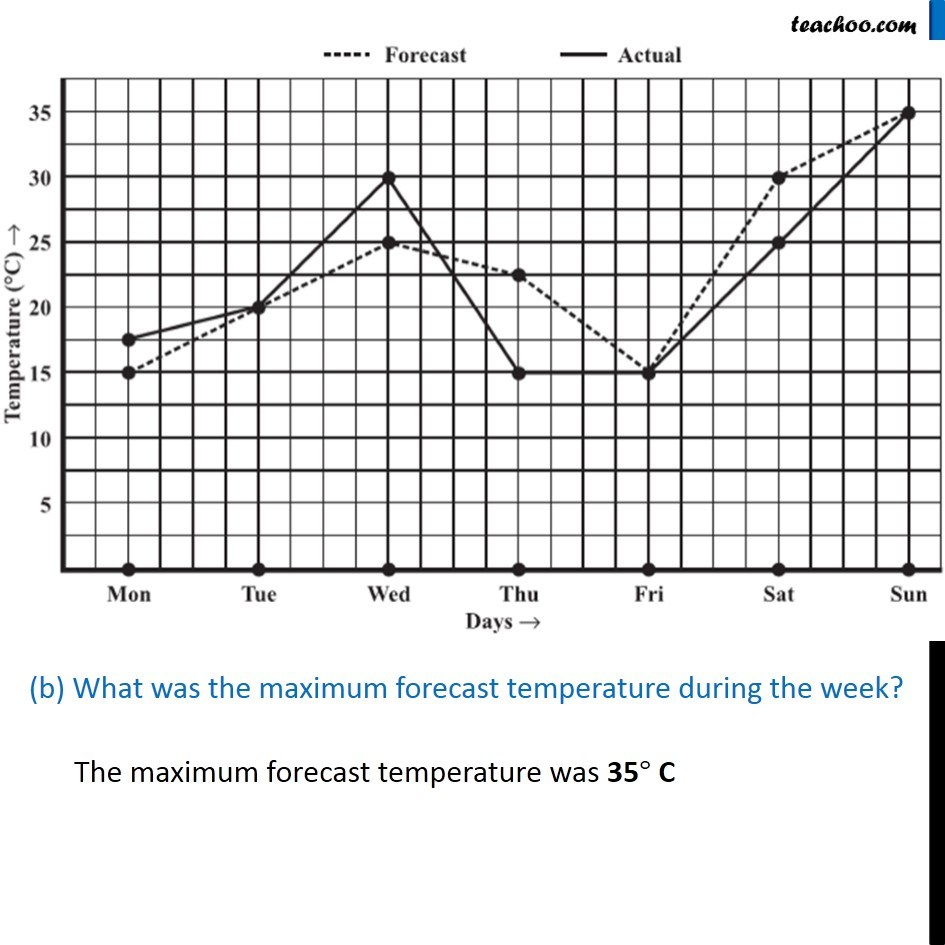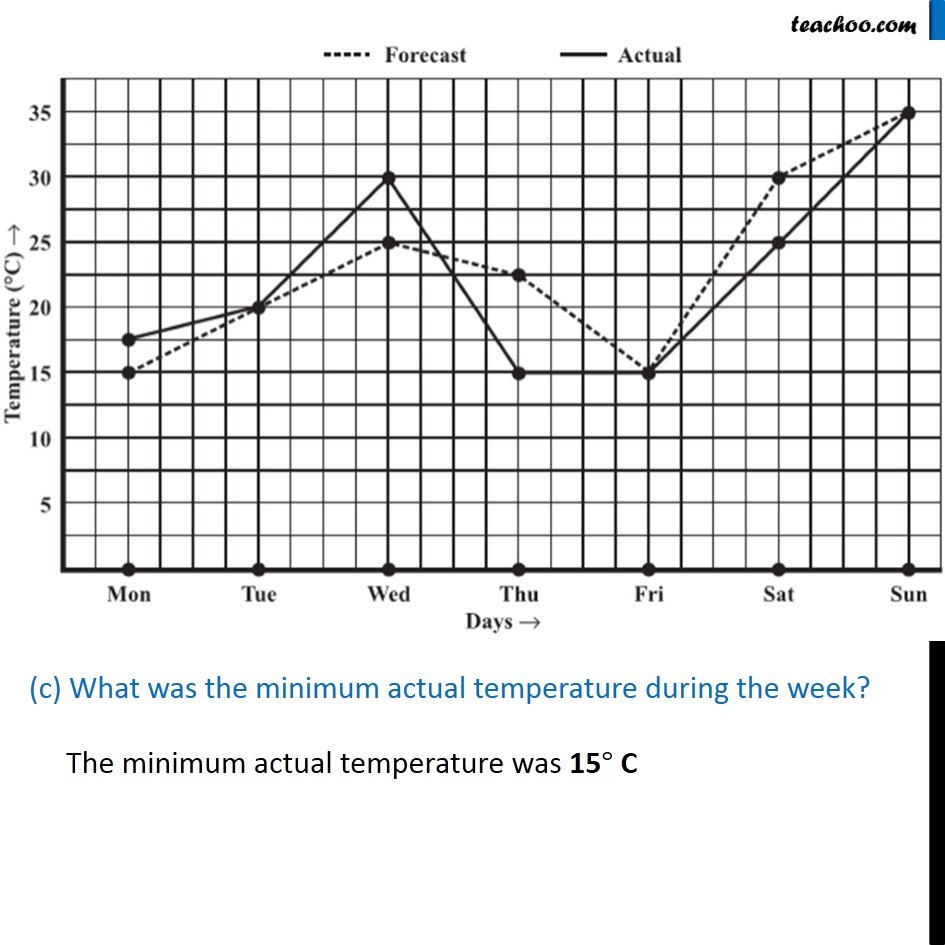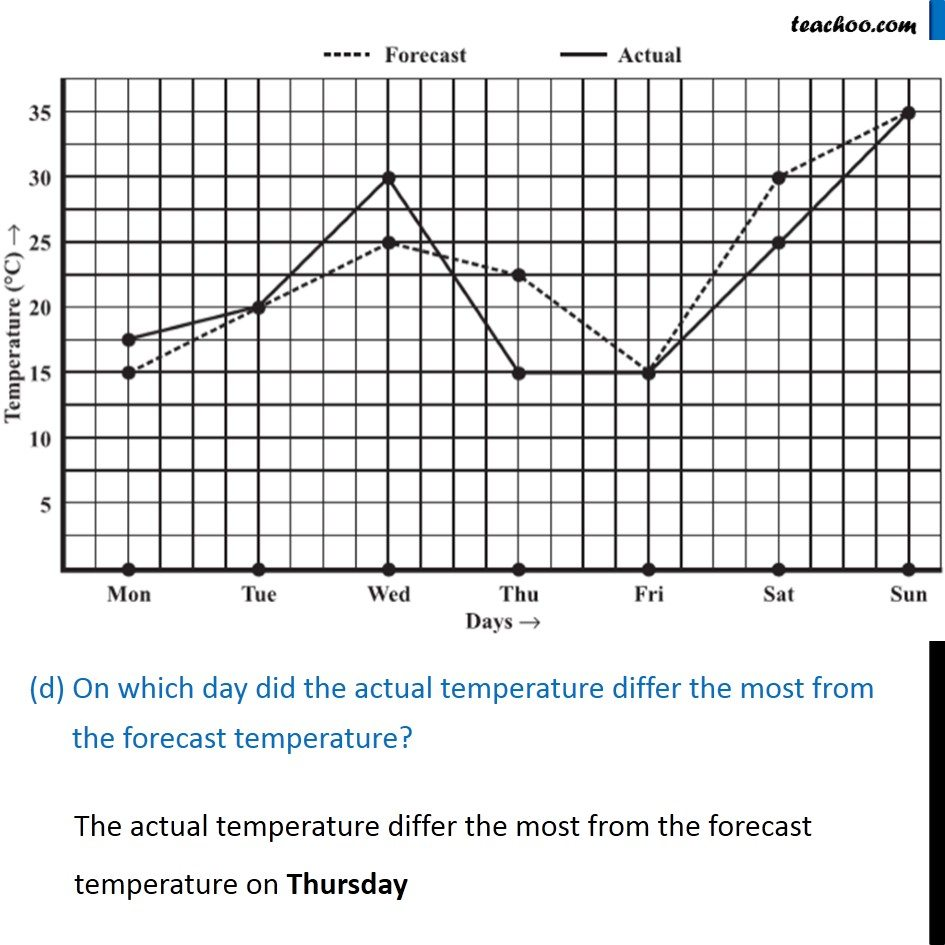1. Chapter 15 Class 8 Introduction to Graphs
2. Serial order wise
3. Ex 15.1

Transcript

Ex 15.1, 4 The following graph shows the temperature forecast the same as the actual temperature for each day of a week. (a) On which days was the forecast temperature the same as the actual temperature ?The forecast temperature is same as the actual temperature on Tuesday, Friday and Sunday. (b) What was the maximum forecast temperature during the week?The maximum forecast temperature was 35° C (c) What was the minimum actual temperature during the week?The minimum actual temperature was 15° C (d) On which day did the actual temperature differ the most from the forecast temperature?The actual temperature differ the most from the forecast temperature on Thursday